Social QuestionUsing only mental calculation, how closely can you approximate 89/99 as a decimal?

Asked by LostInParadise (29298) February 16th, 2013
20 responses
“Great Question” (5)

Hint: If a team has a record of 89 wins out of 99 games, how much can its winning percentage change after playing one more game? Think in terms of weighted averages.Follow this questionSend to a friend!Topics: , ,
Observing members: 0Composing members: 0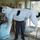just a fast mental calculation I would say about 89.9%, if they win one more it would be 90% and if they lose it would be 89%, but that is a guess.

DrBill (16061)“Great Answer” (2)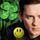I’d call it 89/99 close to 90/100 so 0.90 but it must be a little lower so by using my favorite Newton’s method of error squared. I figure 1 out of 100 squared is .0001 x so I get 0.8999.

LuckyGuy (40286)“Great Answer” (6)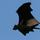Like @LuckyGuy, 89/99 is 90% if you round up to no decimal places. But since it’s not “exactly” 90%, it’s the nearest thing to it.

CWOTUS (26082)“Great Answer” (2)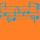… _
0.89 I say this because a number over 9 always gets repeated.

dxs (15160)“Great Answer” (1)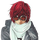@dxs Follow your logic through. How many 9’s are there in the denominator? Thus it should be 0.8989898989…

SavoirFaire (27812)“Great Answer” (1)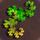0.8989898989898989…

You probably should have picked a different set of numbers, since there is a very simple rule with 9s.

PhiNotPi (12677)“Great Answer” (0)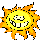I have no idea how to begin figuring. I’d have to ask for help.

Sunny2 (18817)“Great Answer” (0)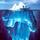89/100 would be exactly 0.89. Making the denominator 1% smaller (99 instead of 100) should make the fraction about 1% larger—an approximation that works because the change is small.
0.89 + 1% = 0.8989.

gasman (11315)“Great Answer” (3)@PhiNotPi 13/17? That’s a toughie. Using my same method as earlier, start by approximating 17 ~= 16.666… = 50/3
That changes the calculation to 13 * 3 / 50 = 39/50 = .39 / .5 = .39+.39 = 0.78.
The real denominator is about 2% bigger than what I used, so the answer should be 2% smaller.
0.78 – 2% = 0.78 – 0.0156
= 0.7644
( The actual answer is 0.7647…)

Hard to do in your head but easier than long division, I think.

gasman (11315)“Great Answer” (2)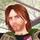Not a chance of me doing maths like that in my head, or even on paper. Nearest I can get without electronic aid is 0.89-plus-a-bit, but I have no idea how big that bit would be.

downtide (23790)“Great Answer” (1)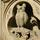Before looking at previous posts or a calculator, I will say 0.9 is close enough for me.

Update 1
OK, I just read the question (not just the headline) and “one more game” makes it more interesting. I shall ponder this.

jaytkay (25795)“Great Answer” (0)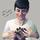Dividing by 9 leaves an interesting pattern: 1/9 = .111…, 2/9 = .222… etc. Dividing by 99 is not much different. It would be .898989…

Mariah (25876)“Great Answer” (1)@PhiNotPi One of the tags is “puzzles,” and puzzles usually have clever answers. I expect that the numbers were chosen because of the trick with nines.

SavoirFaire (27812)“Great Answer” (0)@SavoirFaire I didn’t really understand the reference to “weighted averages.” I assumed that the OP was looking for a different trick, since the trick with nines doesn’t have anything to do with averages (at least I don’t think so).

PhiNotPi (12677)“Great Answer” (0)This is my weighted average approach.
I am going to sometimes refer to 89/99 as x to simplify things.
If a team has won 89 out of 99 games and then loses the next game then the new average of .89 is less than x. Using weighted averages, .89 = .01×0 + .99×89/99.
0=======.89===x. (x – .89) = .01(x – 0) = .01×89/99

If the team wins the next game then the new average is .90 = .01×1 + .99×89/99
x==.9=========1 (x – .9) = .01(1 – x) = .01×10/99

(x – .9) / (x – .89) = 89/10, which is between 8 and 9. Therefore the distance of x from .89 is between 8/9 and 9/10 the distance from .89 to .9, meaning that .8988… < x < .899, using the fact that 8/9 = .888… This guarantees that 89/99 = .899 to 3 places and in fact 89/99 equals .899 to four places, using a calculator.

The same approch can be used for 13/17. Doing a similar approach to the above and having the team either lose the next 3 games or win them all, we have 13/17 located at 13/17 the distance betwen 13/20 = .65 and 16/20 = .8. The distance between .65 and .8 is .15. We can also say that 13/17 is 4/17 the distance going from .8 to .65, so 13/17 = .8 – 4/17 x .15. 4/17 x .15 = .01(60/17) = .01(3 9/17).

9/17 is between .5 and 10/18=5/9=.555… so .764 < 13/17 < .765

LostInParadise (29298)“Great Answer” (1)@PhiNotPi Oh, good point. And @LostInParadise‘s response seems to bear out your assumption.

SavoirFaire (27812)“Great Answer” (0)Just a quick add-on. I used the technique of adding 1 to the denominator and 0 or 1 to the numerator. This can be refined to get better etimates. For 89/99, start by adding 1 to top and bottom to give .9 as an upper bound. Now we can add 1 to the denominator and .9 to the numerator to get an upper bound of .899. One more round gives an upper bound of .89899.

Similarly for the numerator, we could start by noticing that ¾ < 89/99, giving an estmate of .8975 as a lower bound. Now we can use this to get another lower bound of .898975.

13/17 strains mental arithmetic, but we can still apply the same idea. 4/17 < ¼ so 13/17 > ¾. We add 3 to the denominator and to the numerator add 3×¾ = 9/4 = 2.25, giving 15.25 / 20 > .762 as a new lower bound.

For the upper bound , we can start by adding 3 to numerator and denominator, giving .8. Now add 3 to the denominator and 3 x .8 = 2.4 to the numerator, giving 15.4/20 = .77

LostInParadise (29298)“Great Answer” (1)@LostInParadise At that point, It’d just be easier to do the division in my head.

dxs (15160)“Great Answer” (0)With 13/17, I’d probably settle for something a little less precise, since the math would become much easier.

13/17 > 12/18, so 13/17 > 2 / 3
Because of this, we can say that
13/17 > (13+2)/(17+3), so 13/17 > 15/20, which gives us a lower bound of 0.75

13/17 < 3/3
Because of this, we can say that
13/17 < (13+3)/(17+3), so 13/17 < 16/20, which gives us an upper bound of 0.8

0.75 < 13/17 < 0.8

In real life, I would never mentally calculate anything more precise than that.

In general the strategy follows this rule, which also applies when the inequalities are reversed:
If A/B > C/D, then A/B > (A+C)/(B+D)
Assuming that A, B, C, and D are positive numbers.

PhiNotPi (12677)“Great Answer” (0)Also C/D < (A+C)/(B+D). The easiest way to see this is to think of (A+C)/(B+D) as a weighted average of (A/B) and (C/D)
(A+C)/(B+D) = B/(B+D) x A/B + D/(B+D) x C/D

LostInParadise (29298)“Great Answer” (0)